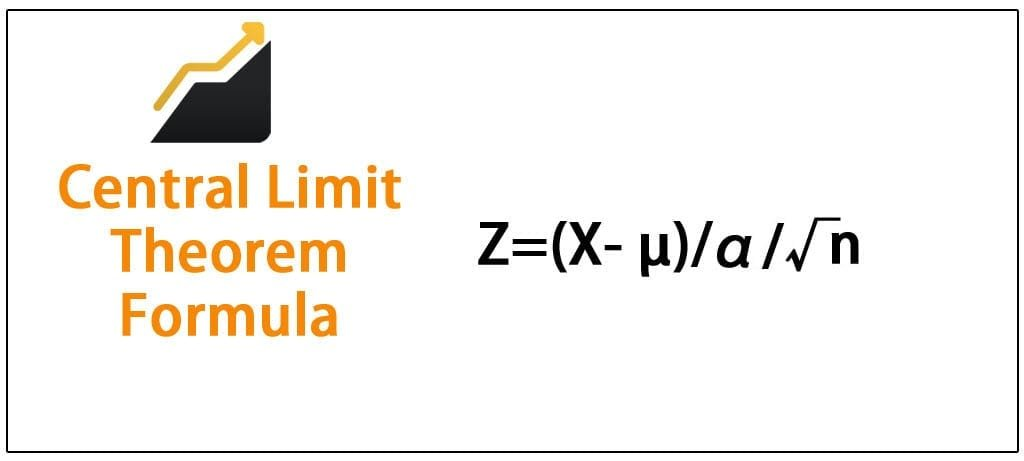Get inspired by the success stories of our students in IIT JAM MS, ISI  MStat, CMI MSc Data Science.  Learn More

# ISI MStat PSB 2012 Problem 5 | Application of Central Limit TheoremThis is a very beautiful sample problem from ISI MStat PSB 2012 Problem 5 based on central limit theorem . Let's give it a try !!

## Problem- ISI MStat PSB 2012 Problem 5

Let $X_{1}, X_{2}, \ldots, X_{j} \ldots$ be i.i.d. $N(0,1)$ random variables. Show that for any $a>0$

$\lim {n \rightarrow \infty} P(\sum_{i=1}^{n} {X_i}^2 \leq a) = 0$

### Prerequisites

Limit

Central Limit Theorem

Normal Distribution

Chi- Square Distribution

## Solution :

$X_{1}, X_{2}, \ldots, X_{j} \ldots$ are i.i.d. $N(0,1)$ random variables .

Let $S_n = \sum_{i=1}^{n} {X_i}^2$ , then $S_n \sim \chi^{2}(n)$ , where $\chi^{2}(n)$ is Chi-Square distribution with n degrees of freedom .

Therefore , $E(S_n)= n$ and $Var(S_n)=2n$ .

In this type of problems obvious thing that would come to our mind is to apply Central Limit Theorem right ! Let's try to apply it .

Now by Lindeberg Levy Central Limit Theorem we can say $\frac{S_n-E(S_n)}{\sqrt{Var(S_n)}}$ = $\frac{S_n-n}{\sqrt{2n}} {\to }^{d} N(0,1)$ , as n approaches infinity.

So, $\lim {n \rightarrow \infty} P(\sum_{i=1}^{n} {X_i}^2 \leq a)$

= $\lim {n \rightarrow \infty} P( \frac{S_n-n}{\sqrt{2n}} \le \frac{a-n}{\sqrt{2n}} )$

= $\lim {n \rightarrow \infty} \Phi(\frac{a-n}{\sqrt{2n}})$

= $\lim {n \rightarrow \infty} \Phi(\frac{a}{\sqrt{2n}}- \sqrt{\frac{n}{2}}) = \Phi(0- \infty)$ (Since $\Phi(x)$ is right continuous ) $= 0$ .

Hence Proved .

## Food For Thought

Let $\{X_{1}: i \geq 1 \}$ be a sequence of independent random variables each having a normal distribution with mean 2 and variance 5.Then $(\frac{1}{n} \sum_{i=1}^{n} x_{i})^{2}$ converges in probability to ?

## Subscribe to Cheenta at Youtube

This is a very beautiful sample problem from ISI MStat PSB 2012 Problem 5 based on central limit theorem . Let's give it a try !!

## Problem- ISI MStat PSB 2012 Problem 5

Let $X_{1}, X_{2}, \ldots, X_{j} \ldots$ be i.i.d. $N(0,1)$ random variables. Show that for any $a>0$

$\lim {n \rightarrow \infty} P(\sum_{i=1}^{n} {X_i}^2 \leq a) = 0$

### Prerequisites

Limit

Central Limit Theorem

Normal Distribution

Chi- Square Distribution

## Solution :

$X_{1}, X_{2}, \ldots, X_{j} \ldots$ are i.i.d. $N(0,1)$ random variables .

Let $S_n = \sum_{i=1}^{n} {X_i}^2$ , then $S_n \sim \chi^{2}(n)$ , where $\chi^{2}(n)$ is Chi-Square distribution with n degrees of freedom .

Therefore , $E(S_n)= n$ and $Var(S_n)=2n$ .

In this type of problems obvious thing that would come to our mind is to apply Central Limit Theorem right ! Let's try to apply it .

Now by Lindeberg Levy Central Limit Theorem we can say $\frac{S_n-E(S_n)}{\sqrt{Var(S_n)}}$ = $\frac{S_n-n}{\sqrt{2n}} {\to }^{d} N(0,1)$ , as n approaches infinity.

So, $\lim {n \rightarrow \infty} P(\sum_{i=1}^{n} {X_i}^2 \leq a)$

= $\lim {n \rightarrow \infty} P( \frac{S_n-n}{\sqrt{2n}} \le \frac{a-n}{\sqrt{2n}} )$

= $\lim {n \rightarrow \infty} \Phi(\frac{a-n}{\sqrt{2n}})$

= $\lim {n \rightarrow \infty} \Phi(\frac{a}{\sqrt{2n}}- \sqrt{\frac{n}{2}}) = \Phi(0- \infty)$ (Since $\Phi(x)$ is right continuous ) $= 0$ .

Hence Proved .

## Food For Thought

Let $\{X_{1}: i \geq 1 \}$ be a sequence of independent random variables each having a normal distribution with mean 2 and variance 5.Then $(\frac{1}{n} \sum_{i=1}^{n} x_{i})^{2}$ converges in probability to ?

## Subscribe to Cheenta at Youtube

This site uses Akismet to reduce spam. Learn how your comment data is processed.

### 2 comments on “ISI MStat PSB 2012 Problem 5 | Application of Central Limit Theorem”

1.MathGuy says:

We can solve this problem using only the Strong Law (SLLN). We do not need the CLT here.
Using Reverse Fatou's lemma, we have
$\limsup \mathbb{P}(\frac{1}{n}\sum_{i=1}^n X_i^2 \leq \frac{a}{n}) \leq \mathbb{P}(\limsup \frac{1}{n} \sum_{i=1}^n X_i^2 \leq 0) = \mathbb{P}(1\leq 0)=0.$

1.Pravat Kumar Hati says:

It's okay if you want to solve in that way .

### Knowledge Partner